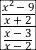# How do you simplify a rational expression within a rational expression?

## Question

How do you simplify ?

## Solution

This is a complex fraction, a fraction with fractions in it. To be simplified it needs to have just one numerator and just one denominator.Treat this like the division problem it is. For fractions, division works like this: . . In other words, flip the bottom fraction and multiply. Factor where possible so you can see any places where cancellation is possible. The x – 3 term shows up in both numerator and denominator. Cancel it.

Are we finished? Maybe. That business in the question about being defined? The expression – either the original or the simplified version – is undefined for any value of xthat would make any of the three denominators in the original expression –  ,  , and  — equal to zero. The words “for which it is defined” just mean you can assume you’re not dealing with any of those values of x.

This question is about rational expressions, complex fractions, and domain constraints.